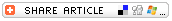## Search formBack to the article
Magic SumSubjects

• Mathematics

Grade

• 3-5
• 6-8

Brief Description

Students learn a "magic" trick as they practice two-digit addition.

Objectives

Students practice addition and study the relationships between numbers.

Keywords

addition, magic

• paper and pencils
• calculators (optional)

Lesson Plan

• Have students draw five boxes in a column on their papers.
• Ask each student to pick a two-digit number and write that number in the first box on their papers. Tell students you will predict their magic sums just from knowing the number in the first box on their papers -- before any other numbers are chosen.
• Have each student tell you the number he or she wrote in the first box. Record the students' names and numbers on a separate piece of paper. To find each student's magic sum, add 198 to the number they wrote in the first box. (Find the number more quickly by adding 200, then subtracting 2.) Record those sums on your paper, and put the paper out of your reach.
• Ask a student to pick any two-digit number. Have all students write the number that student called out in the second box on their papers.
• Tell students that you will pick the number for the third box on their papers. (To choose a number for that box, subtract the number in the second box from 99.) Pretend to choose a number at random and have all the students write your number in the third box.
• Ask another student for a two-digit number, and have all students write that number in the fourth box on their papers.
• Tell students that you will pick a two-digit number for the fifth box. (Subtract the number in the fourth box from 99.) Pretend to pick a number at random, and have students write that number in the fifth box.
• Ask students to add the numbers in their boxes to find the magic sum.
• Display your "mathemagic" powers by having a student read the name of each student and the magic sum you predicted for that student.
• Challenge students to discover the secret of the trick.

Students should discover that the sum of the second and third boxes is 99, and so is the sum of the fourth and fifth boxes; therefore, the magic sum is the number in the first box +99 +99 or the number in the first box +198 or the number in the first box + 200 - 2.

Assessment

N/A

Lesson Plan Source

Teaching Children Mathematics

National Standards